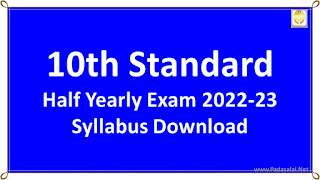No.1 Educational Website

15. BUSINESS MATHS — SYLLABUS (2022 - 23)

APPLICATIONS OF MATRICES AND DETERMINANTS :
1.1 Rank of a Matrix

1.2 Cramer's Rule

1.3 Transition Probability Matrices

INTEGRAL CALCULUS -I

2.1 Indefinite Integrals

2.2 Definite Integrals

MONTHLY TEST (JUNE PORTION ONLY)

INTEGRAL CALCULUS - II

3.1 The area of the region bounded by the curves

3.2 Applications of Integration in Economics and Commerce
DIFFERENTIAL EQUATIONS:

4.1 Formation of ordinary differential Equations

4.2 First order and first degree differential equations

FIRST MID TERM TEST & MONTHLY TEST (JUNE & JULY PORTIONS)

DIFFERENTIAL EQUATIONS:

4.3 Second Order First Degree linear differential equations with constant co-efficients
NUMERICAL METHODS:

5.1 Finite differences

5.2 Interpolation

MONTHLY TEST (AUGUST PORTION ONLY)

RANDOM VARIABLE AND MATHEMATICAL EXPECTATION

6.1 Random Variable
6.2 Mathematical Expectation
QUARTERLY EXAMINATION (JUNE TO SEPTEMBER PORTIONS)